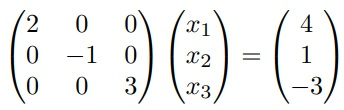# Python code for backward substitution method for solving the linear system

19

## Description

This function uses the backward substitution method for solving the linear system Ax = b, where A is an upper triangular matrix b is a known vector and n is the dimension of the problem.

## Example :```A = np.diag([2, -1, 3])

b = np.array([4, -1, 3])

Final result of the python code

x =
[[ 2.]
[ 1.]
[ 1.]]```

## Reviews

There are no reviews yet.

SKU: 2PYUPGZLISY3 Category: